# Diagonal of a Polygon Formula

• Last Updated : 01 Feb, 2022

Polygon can be defined as a closed figure which is formed by joining the straight lines. So it is easy to see that to make a polygon at least three lines are needed. There are polygons known with different names depending on the number of lines by which it is formed.

### Diagonals of a Polygon

The diagonal of a polygon can be defined as a line that joins the end of two adjacent sides of any polygon and it is generally inside a polygon.

Example: A polygon formed from 3 lines is Triangle, Polygon with 4 lines is a quadrilateral, Polygon with 5 lines is a pentagon, and so on.

Formula of Diagonals of a polygon

Diagonals = (n × (n – 3))/2

Where n is the number of sides of a polygon

Proof

For making a diagonal in a polygon we need two vertices. Let’s consider an N-sided polygon, now each vertex can be connected to the other in nC2 different ways but in this, the number of sides which is n is taken twice so subtract n from the total number of ways.

Hence number of diagonals = nC2 – n

= (n!)/(n – 2)! × (2!) – n

= n(n – 1)(n – 2)!/(n – 2)! × (2!) – n

= n(n – 1)/2 – n

= (n(n – 1) – 2n)/2

= n(n – 3)/2

Example 1: Triangle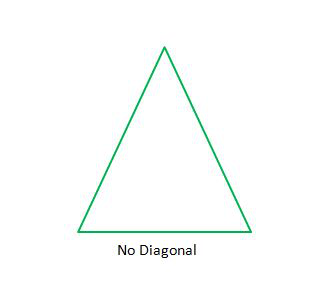Take triangle and find the number of diagonals in it.

So a triangle has 3 sides so n = 3

Using formula, diagonals = (n × (n – 3))/2

Put n =3

Diagonals = (3 × (3 – 3))/2

= 0

Hence a triangle has zero diagonals.

Example 2: Square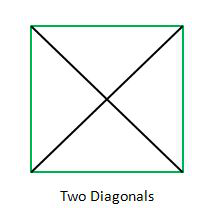Take Square and find the number of diagonals in it.

So a Square has 4 sides so n = 4

Using formula, diagonals = (n×(n-3))/2

Put n = 4

Diagonals = (4 × (4 – 3))/2

= 2

Hence a square has two diagonals.

Example 3: Pentagon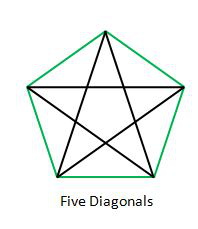Take pentagon and find the number of diagonals in it.

So a pentagon has 5 sides so n = 5

Using formula, diagonals = (n × (n – 3))/2

Put n = 5

Diagonals = (5 × (5 – 3))/2

= 5

Hence a pentagon has five diagonals.

### Sample Problems

Question 1: How many diagonals do a hexagon has, find using the diagonal of a polygon formula.

Solution: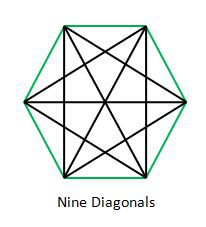Hexagon is a polygon that is formed by six straight lines

So a hexagon has 6 sides so n = 6

Using formula, diagonals = (n × (n – 3))/2

Put n = 6

Diagonals = (6 × (6 – 3))/2

= 9

Hence a hexagon has nine diagonals.

Question 2: There are 20 diagonals in a polygon, find a number of sides it has?

Solution:

Using diagonals formula = (n × (n – 3))/2

So 20 = (n × (n – 3))/2

20 × 2 = (n × (n – 3))

40 = n2 – 3 × n

n2 – 3 × n – 40 = 0

n2 – 8n + 5n – 40 =0

n(n – 8) + 5(n- 8) = 0

(n – 8)(n + 5) = 0

So n = 8

Hence the polygon is the octagon.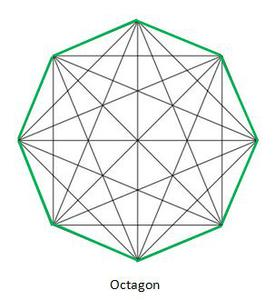Question 3: How many diagonals do a decagon has, find using the diagonal of a polygon formula.

Solution:

A decagon has 10 sides so n = 10

Using formula, diagonals = (n × (n – 3))/2

Put n = 10

Diagonals = (10 × (10 – 3))/2

= 35

Hence a decagon has 35 diagonals.

Question 4: There are 27 diagonals in a polygon, find the number of sides it has?

Solution:

Using diagonals formula = (n × (n – 3))/2

So 27 = (n × (n – 3))/2

27 × 2 = (n × (n – 3))

54 = n2 – 3 × n

n2 – 3 × n – 54 = 0

n2 – 9n + 6n – 54 =0

n(n – 9) + 6(n- 9) = 0

(n – 9)(n + 6) = 0

So n = 9

Hence the polygon is Nonagon.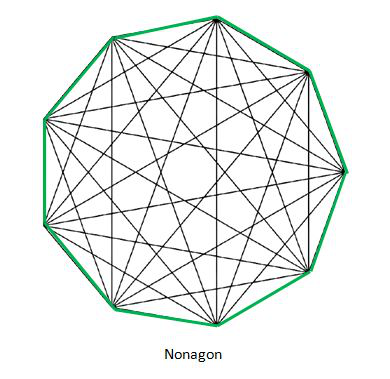Question 5: How many diagonals does a polygon have if sides are 20?

Solution:

Put n = 20 in diagonals formula

Diagonals = (20 × (20 – 3))/2

= 170

Hence there will be 170 diagonals in a 20 sided polygon.

Question 6: There are 405 diagonals in a polygon, find the number of sides it has?

Solution:

Using diagonals formula = (n × (n – 3))/2

So 405 = (n × (n – 3))/2

405 × 2 = (n × (n – 3))

810 = n2 – 3 × n

n2 – 3 × n – 810 = 0

n2 – 30n + 27n – 810 =0

n(n – 30) + 27(n – 30) = 0

(n – 30)(n + 27) = 0

So n = 30

Hence the polygon has 30 sides.

Question 7: How many diagonals does a polygon have if sides are 40?

Solution:

Put n = 40 in diagonals formula

Diagonals = (40 × (40 – 3))/2

= 740

Hence there will be 740 diagonals in a 40 sided polygon.

My Personal Notes arrow_drop_up